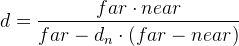# Encoding depth and confidence

A depthmap is serialized as a set of XMP properties. As part of the serialization process, the depthmap is first converted to a traditional image format. The encoding pipeline contains three steps (see Figure 2):

• Convert from the input format (e.g. float or int32 values) to an integer grayscale image format, e.g. bytes (8 bits) or words (16-bit).
• Compress using a standard image codec, e.g. JPEG or PNG.
• Serialize as a base64 string XMP property.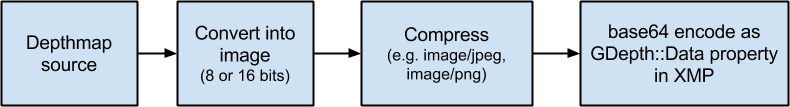Figure 2. Description of the depthmap encoding pipeline.

The pipeline can be lossless or lossy, depending on the number of bits of the original depthmap and the number of bits used to store it, e.g. 8 bits for an JPEG codec and 8 or 16 bits for a PNG codec.

Two different formats are currently supported: RangeLinear and RangeInverse. RangeInverse is the recommended format if the depthmap will lose precision when encoded, e.g. when converting from float to 8-bit. It allocates more bits to the near depth values and fewer bits to the far values, in a similar way to how the z-buffer works in GPU cards.

If the depthmap has an attached confidence map, the confidence map is also converted to a traditional image format using a similar pipeline to the one used for depth. The confidence map is always encoded using the RangeLinear format, with the confidence range assumed to be [0, 1].

### RangeLinear

Let d be the depth of a pixel, and near and far the minimum and maximum depth values considered. The depth value is first normalized to the [0, 1] range as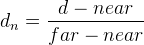then quantize to 8 or 16 bits as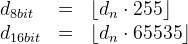Conversely, given the quantized depth d8bit, one can recover depth d as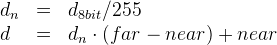### RangeInverse

Let d be the depth of a pixel, and near and far the minimum and maximum depth values considered. The depth value is first normalized to the [0, 1] range as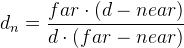then quantize to 8 or 16 bits asConversely, given the normalized depth dn, one can recover depth d as